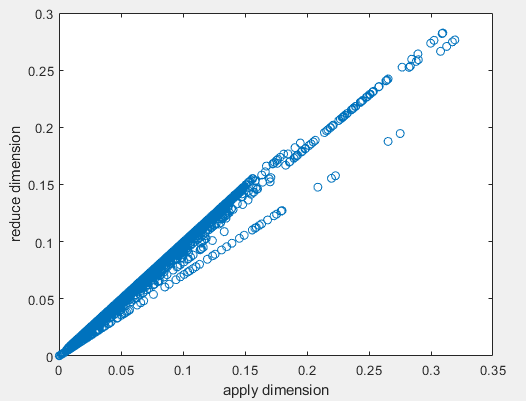# Which is the right function to apply sd

Hi
I am trying to calculate the temporal standard deviation of the NIR reflectance from Sentinel-2, in order to use the obtained product to create a mask. I have found two ways to do so:
The operation:
s=datacube.apply_dimension(process=‘sd’,dimension=‘t’)
and the operation:
s=datacube.reduce_dimension(reducer=‘sd’,dimension=‘t’)

the second one is more useful to me, since it remove the time dimension after the calculation and therefore there is no need to apply the “drop_dimension” operation after this one, but when I tried to download the results, the two maps did not coincide:Which one is the function to be used?

You should indeed use `reduce_dimension`! Using `reduce_dimension` with `'sd'` as reducer over time will compute the standard deviation for each pixel timeseries and drop/remove the temporal dimension.

The `apply_dimension` process is a bit different and suitable for other scenarios. Please see here the corresponding documentation:
openEO API docs: openEO API Processes
Pyton client docs: API — openEO Python Client 0.10.1a1 documentation

I thought so, but I tried to download the datacube and performed the sd externally: there seems to be an issue with the “reduce_dimension” operation.

Thanks for the very nice report!
We already knew that we could optimize this, but this makes it even higher priority.
Logged it here: